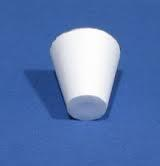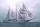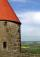# Truncated cone 5

The height of a cone 7 cm and the length of side is 10 cm and the lower radius is 3cm. What could the possible answer for the upper radius of truncated cone?

Correct result:

r1 =  10.1414 cm

#### Solution:We would be pleased if you find an error in the word problem, spelling mistakes, or inaccuracies and send it to us. Thank you!Tips to related online calculators
Do you want to convert length units?
Pythagorean theorem is the base for the right triangle calculator.

#### You need to know the following knowledge to solve this word math problem:

We encourage you to watch this tutorial video on this math problem:

## Next similar math problems:

• Center traverseIt is true that the middle traverse bisects the triangle?
• Is right triangleDecide if the triangle XYZ is rectangular: x = 4 m, y = 6 m, z = 4 m
• Isosceles triangleCalculate the area of an isosceles triangle, the base of which measures 16 cm and the arms 10 cm.
• SatinSanusha buys a piece of satin 2.4 m wide. The diagonal length of the fabric is 4m. What is the length of the piece of satin?
• Median in right triangleIn the rectangular triangle ABC has known the length of the legs a = 15cm and b = 36cm. Calculate the length of the median to side c (to hypotenuse).
• Right angleIf a, b and c are two sides of a triangle ABC, a right angle in A, find the value on each missing side. If b=10, c=6
• SailingSolve the following problem graphically. The fishing boat left the harbor early in the morning and set out to the north. After 12 km of sailing, she changed course and continued 9 km west. Then When she docked and reached the fishing grounds she launched
• Spruce heightHow tall was spruce that was cut at an altitude of 8m above the ground and the top landed at a distance of 15m from the heel of the tree?
• CablewayCableway has a length of 1800 m. The horizontal distance between the upper and lower cable car station is 1600 m. Calculate how much meters altitude is higher upper station than the base station.
• DistanceWha is the distance between the origin and the point (18; 22)?
• Euclid 5Calculate the length of remain sides of a right triangle ABC if a = 7 cm and height vc = 5 cm.The double ladder is 8.5m long. It is built so that its lower ends are 3.5 meters apart. How high does the upper end of the ladder reach?Right isosceles triangle has an altitude x drawn from the right angle to the hypotenuse dividing it into 2 unequal segments. The length of one segment is 5 cm. What is the area of the triangle? Thank you.The double ladder shoulders should be 3 meters long. What height will the upper top of the ladder reach if the lower ends are 1.8 meters apart?How far can you see from the ship's mast, whose peak is at 14 meters above sea level? (Earth's radius is 6370 km).The cone roof of the church has a diameter of 3m and a height of 4m. What is the size of the side edge of the church roof (s) and how much sheet will be needed to cover the church roof?The radius of the base of a right circular cone is 14 inches and it's height 18 inches. What is the slant height?